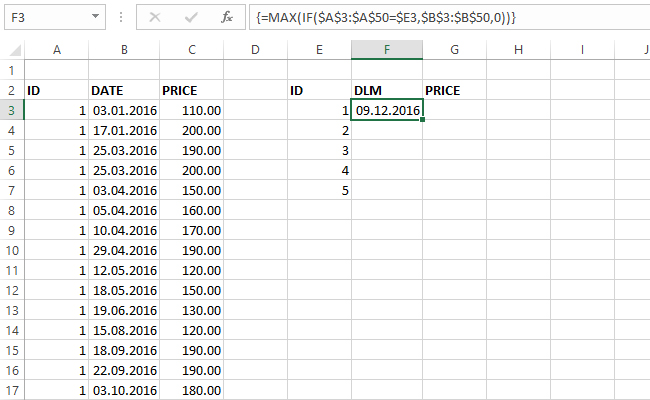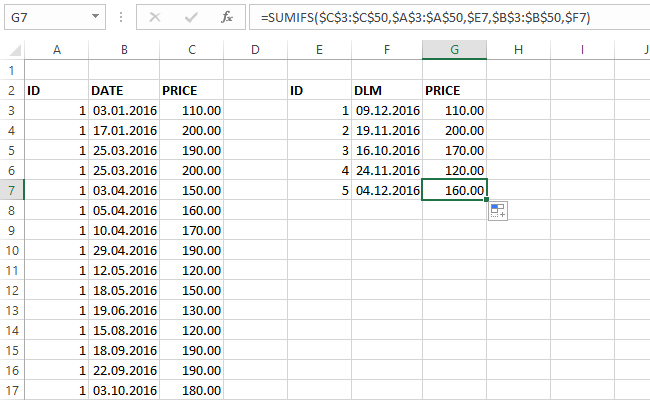# Current prices

The catalog is a list of items associated with prices that and, by rule, it should not be changed more than once a day. In practice it is formed by consecutive adding codes of items, dates and prices in the table. How to find the last (current) price? Although at first glance it seems simple, this can be a considerable brain teaser. Also, this is an excellent demonstration of the practical application of formulas containing arrays and one of the “recipes” you will surely like.

To begin with, we need a table with three columns: ID, DATE (price changes) and PRICE. We should fill them with random data and then sort in ascending order according to two criteria: ID and DATE. Then we should create another table with columns: ID, DLM (date of last modification) and PRICE. This is the table where we’re going to store results.

First, it is necessary to determine the dates of last modification for each item. They are important because the date of the last modification indicates the current price. For this purpose, we will insert this array-formula:

=MAX(IF(\$A\$3:\$A\$50=\$E3,\$B\$3:\$B\$50,0))

This formula compares the value of the first column (ID) with the ID in second table, and if the condition is met takes result from the second column (DATE). When we nest this formula within MAX function, and complete it as an array (CTRL + SHIFT + ENTER) will get the maximum value of the second column to the given criteria, i.e. date of last modification for current item.

{=MAX(IF(\$A\$3:\$A\$50=\$E3,\$B\$3:\$B\$50,0))}

Formula should be completed as an array, otherwise we won’t get correct result. Since value in cell E3 is marked as \$E3 (fixed column), just by copying remaining cells we will get the dates of last price changes.Now we only need to find current price. For this purpose we will use the function SUMIFS. Its first argument should be a column with the prices in price list, and as a condition for comparison we will use two columns in result table which we compare with the reference columns in the price list. Now we enter following formula:

=SUMIFS(\$C\$3:\$C\$50,\$A\$3:\$A\$50,\$E3,\$B\$3:\$B\$50,\$F3)

By copying formula to the remaining cells we’ll get current prices for all other items.